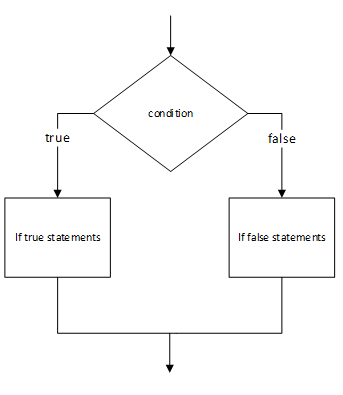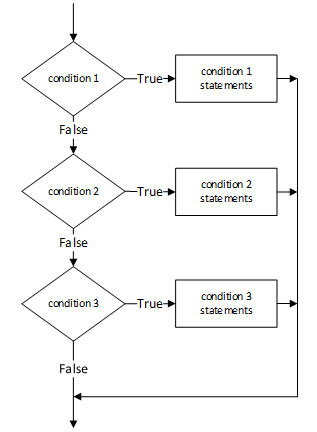## If Else Conditional Statement In C Language

### if statement :

The if statement is the most general method for allowing conditional execution in C.

Syntax
```if  ( condition )
statement body ;
else
statement body ;```

or just :

Syntax
```if ( condition )
statement body ;```In the first more general form of the statement one of two code blocks are to be executed. If the condition evaluates to TRUE the first statement body is executed otherwise for all other situations the second statement body is executed.

In the second form of the statement the statement body is executed if the condition evaluates to TRUE. No action is taken otherwise.

For Example : Program to perform integer division avoiding the division by zero case.

CODE/PROGRAM/EXAMPLE
```#include <stdio.h>
void main()
{
int numerator, denominator ;

printf( "Enter two integers as follows numerator, denominator :" );
scanf( "%d,  %d", &numerator, &denominator  ) ;

if ( denominator != 0 ){
printf( "%d / %d = %d \n", numerator, denominator, numerator / denominator );
}
else{
printf( "Invalid operation - unable to divide by zero \n” );
}
}```

As with all other control statements the statement body can also involve multiple statements, again contained within curly braces.

Example :- Program to count the number of occurrences of the letter 'a' in an input stream of characters terminated with a carriage return.

CODE/PROGRAM/EXAMPLE
```#include <stdio.h>
void main()
{
int count = 0, total = 0 ;
char ch ;

while (  ( ch = getchar() ) != 13 )    // 13 is ASCII value for
//carriage return
{
if ( ch == ‘a’ )
{
count ++ ;
printf( "\n Retrieved letter ‘a’ number %d\n", count ) ;
}
total ++ ;
_flushall() ;
}

printf( "\n\n %d letters a typed in a total of %d letters.",
count, total ) ;
}```

### Nested if statements:

if - else statements like all other decision or iteration statements in C can be nested to whatever extent is required. Care should be taken however to ensure that the if and else parts of the statement are matched correctly -- the rule to follow is that the else statement matches the most recent unmatched if statement.Syntax
```For Example :-
if ( x > 0 )
if ( x > 10 )
puts ( " x is greater than zero and also greater than 10 ");
else
puts ("x is greater than zero but less than or equal to 10");```

The else clause matches the most recent unmatched if clause, if ( x > 10 ). For more clarity the above section could be rewritten as follows using curly braces with no execution penalty :-

Syntax
```if ( x > 0 )
{
if ( x > 10 )
puts ( " x is greater than zero and also greater than 10 ");
else
puts ( "x is greater than zero but less than or equal to 10 ");
}```
#if_-_Else_conditional_statement_in_C_language #if_else_c #if_else_in_c #control_statements_in_c #if_statement_in_c #if_else_statement_in_c #if_statement_in_c #Nested_if_statements_in_c #

### (New page will open, for Comment)

Not yet commented...